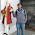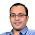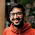### Determinant of Binary Matrix

Source: Introduced to me by Sudeep Kamath (PhD Student, UC at Berkeley, EE IITB Alumnus 2008)

Problem:

An N by N matrix M has entries in {0,1} such that all the 1's in a row appear consecutively. Show that determinant of M is -1 or 0 or 1.

Disclaimer:
I could not solve it but I have an awesome solution sent by Pritish Kamath (MSR Research Assistant, CSE IITB Alumnus 2012)

Update (2/4/2013):
Solution posted by Amol Sahasrabudhe (IITB 2004 Alumnus, Ex-Morgan Stanley Quant Associate, Deutsche Bank Quant Associate) and Piyush Sao (EE IITM Alumnus, Georgia Tech Grad Student) in comments! Thanks a ton. I have posted the solution provided by Pritish Kamath (MSR Research Assistant, CSE IITB Alumnus 2012). All three solutions are essentially the same.

1.First post so pardon the formatting.Use induction. Assume the property be true for all (N-1)x (N-1)matrices . It is trivially true for 2x2 matrices. let S={R_i} be set of rows with a 1 in first column. If this set is empty then determinant is 0 and we are done. Other wise arrange the rows in S by the number of 1s in the row so that R_1 has less 1's than R_2 and so on. Subtract R_1 from all rows in S. Since all the ones are together, this operation cannot introduce a -1 in the matrix and preserves determinant. Now expand along the first column which has only one `1'. It is easy to see that the cofactor(Minor?) satisfies the binary property so that by induction determinant is either +1,-1 or 0.

2.I think this can be proved using induction and a bit of LU factorization type procedure. My arguments goes as follows.

For N =1 , it is trivial.

With strong induction hypothesis, we assume that it is also true for N=1,2... k-1.

Now for N=k, and any arbitrary matrix A, with given property, We apply following operations which keeps absolute value of determinant invariant.

1. Permute rows of A such that, rows in which streak of 1's start at first position, are in the begining

example :
[ 0, 1
1, 1]

becomes
[ 1, 1
0, 1]

2. And, among those rows, one with least number of 1's , comes first
i.e.

example :
[ 1, 1, 1
1, 1, 0
..]

becomes
[ 1, 1, 0
1, 1, 1
..]

3. Now among all those rows where streak of 1's start at first position, Subtract first row from it

example :
[ 1, 1, 0
1, 1, 1
..]

becomes
[ 1, 1, 0
0, 0, 1
..]

4. After this operation, Only in first row would be the only row with 1 in the first column, and the lower right N-1*N-1 block would be again a block with the stated property, hence by priciple of mathematical induction, determinant would be either of 1,0 or -1, so would be of the original matrix

3.Here's a crude method for 3X3 matrix: by disproving contradiction

Take a 3X3 matrix M. In order for it to be not equal to +1,0,-1, the co-factor of M should be either 0 or -1. Other case will be when co-factor of one of either M or M is 0 and relevant are assigned to cofactors of M.
In the first case:
If cofactor of M is zero, then last 2 elements in first and last column are zero or one; or one of them is all zero and the other one is all one. the condition is imposed that 1's will not be discontinuous in the matrix. After formulating the required matrix, we can see that determinant of matrix is not 2 or -2.
If cofactor of M is -1, the cofactors of M and M are -1, so the determinant becomes -1.

1.I am not able to understand your solution. Please explain clearly. Thanks

4.Solution provided by Pritish Kamath:

[Proof by induction]
Base case : The result is clearly true for 1x1 matrices.

Induction step : Assume that the result is true for (N-1)x(N-1) matrices with this property.

For an NxN matrix, there are two possibilities.
(a) M does not have any row with 1 as it's first column entry
In this case the determinant is clearly zero.

(b) M has atleast 1 row with 1 as it's first column entry
Take one such row and interchange it with the first row of M and subtract this row from every other row which has 1 as it's first column entry. The resulting matrix has the property that for any row, all it's non-zero entries appear consecutively and all of them are either +1 or all of them are -1. Multiply -1 to all the rows which have -1 entries. Also, note that the (1,1) entry is 1, and it is the only non-zero entry in the entire first column. Hence the determinant is equal to the determinant of the minor of (1,1). Because we have multiplied by -1's here and there, the sign of the determinant might change, but it'll either be +1 or -1.

1.Consider Matrix [[1,0,1],[1,1,0],[0,1,0]] now if you subtract 2-1 than matrix will be [[1,0,1],[0,1,-1],[0,1,0]] now how will you make it binary array?

5.Thanks Amol, Piyush and Pritish (posted by me) for correct solution! :)

6.In case you are interested in a non-inductive proof: Say the rows of this matrix are R_1,R_2,...,R_n. They have the property that whenever 1's occur in any R_i, they occur consecutively. Denote the position of the first 1 in row R_i by l_i. It is clear that the row R_i gets uniquely determined by the value of l_i and vice versa. Of course 1 <= l_i <= n. If any l_i=n for some R_i, then clearly det=0. Further if there exist R_i,R_j such that l_i=l_j then det=0 (det is a multilinear function of rows). Suppose the determinant is non-zero. Note that we then have l_i \neq l_j for every i,j and that 1 <= l_i < n for every i. Thus there is a permutation p, such that 1 <= l_p(1) < l_p(2) < ... < l_p(n) < n (basically we are re-ordering the rows in increasing value of l_i's). Since the l_i's are integral it is immediately obvious that 1=l_p(1) and that l_p(i+1)=l_p(i)+1. Since the rows were permuted by p, depending on sign of p, we would get det either 1 or -1

7.Aurko's solution expanded :
If there is any row which does not contain any 1, then the determinant is 0. Otherwise in every row, there is at least one 1. Say the rows of this matrix are R_1,R_2,...,R_n. They have the property that whenever 1's occur in any R_i, they occur consecutively. Denote the position of the first 1 in row R_i by l_i and last 1 by r_i. It is clear that the row R_i gets uniquely determined by the tuple (l_i,r_i) and vice versa. Of course 1 <= l_i <= n and 1 <= l_i <= n. Further if there exist R_i,R_j such that l_i=l_j and r_i=r_j then det=0 (det is a multilinear function of rows).
If there exist R_i,R_j such that l_i=l_j and r_i>r_j, then by transforming R_i to R_i-R_j we obtain a new matrix whose determinant is same as original and the row R_i is now associated with tuple (1+r_j,r_i). By repeating this process, we obtain a matrix in which we have l_i \neq l_j for every i,j and that 1 <= l_i <= n for every i. Now by Aurko's argument, the determinant would either be 1 or -1.